# Two trains

There are two trains running the same distance. 1st train will travel it in 7 hours 21minutes. 2nd the train will travel 5 hours 57minutes and 34 seconds and it is 14 km/h faster than the first train. What are speeds of trains and how long is this railway?

a =  60 km/h
b =  74 km/h
s =  441 km

### Step-by-step explanation:

b=a+14
a•(7+21/60)=b•(5+57/60+34/3600)

a-b = -14
26460a-21454b = 0

a = 59.999201
b = 73.999201

Our linear equations calculator calculates it.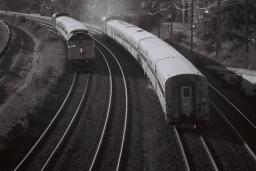Did you find an error or inaccuracy? Feel free to write us. Thank you!Tips to related online calculators
Do you have a linear equation or system of equations and looking for its solution? Or do you have a quadratic equation?
Do you want to convert velocity (speed) units?
Do you want to convert time units like minutes to seconds?

## Related math problems and questions:

• TrainsFrom station 130 km away started passenger train and after 2.2 hours after the express train, which travels 37 km an hour more. Express train finish journey 7 minutes early. Calculate the average speed of this two trains.
• Two trains meetFrom A started at 7:15 express train at speed 85 km/h to B. From B started passenger train at 8:30 in the direction to A and at speed 55 km/h. The distance A and B are 386 1/4 km. At what time and at what distance from B the two trains meet?
• Train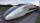A passenger train traveled for 2 hours 74 km. 3.1 hours after its departure started fast train and caught it on 186 km. How many km/h is different its average speeds?
• Car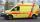The car drove some distance in 3 hours and 20 minutes. If it increases speed by 10 km/h, it goes this distance in 2.5 hours. Calculate the distance.
• TruckIn 7 hours started from town Krnov truck at speed 40 km/h. Passenger car started against it in 8 hours 30 minutes from the city of Jihlava at speed 70 km/h. Distance between this two cities is 225 km. At what time and at what distance from Krnov this two
• Cars 2Opava is 360 km distance from Prešov. At 8:00 hour, two cars started against each other from this cities at average speeds 88 km/h and 121 km/h. What hour will it meet and what distance it's travel if the car started from Prešov is faster?
• Truck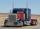Truck went for 4 hours and 30 minutes. If truck increase speed by 11 km/h, went same distance in 2 hours. Calculate this distance.
• Train 2The train slowed down from 90 km/h to 72 km/h in 5 seconds. How long track travel?
• Two cars 2Two cars started from two positions 87 km distant at the same time in opposite directions at speeds 81 km/h and 75 km/h. What was the distance between them after 2 hours 50 minutes of driving.
• Tourists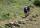From the cottage started first group of tourists at 7:00 AM at speed 4 km/h. The second started after them 21 minutes later at speed 6 km/h. How long and how many kilometers from the cottage will catch the first group?
• Otto and Joachim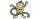Otto and Joachim go through the woods. After some time Otto tire and make 15 minutes stop. Joachim meanwhile continues at 5 km/h. Otto when he set off again, first running speed of 7 km/h, but it keep only 30 sec and 1 minute must continue at 3 km/h. This
• Two trainsThrough the bridge, long l = 240m, the train passes through the constant speed at time t1 = 21s. A train running along the traffic lights at the edge of the bridge passes the same speed at t2 = 9s. a) What speed v did the train go? b) How long did it take
• Journey 5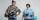A man has to do a journey of 84km in 3 hours. He travels the first 30km at 20km/hr. At what rate must he travel the remaining distance to complete his journey on time?
• Train from PragueThe first train from Prague started at 8:00 hour at 40 kilometers per hour. Train from Ostrava started at 9:20 at 80 km per hour. How many hours and how far from cities with trains meet if the distance of cities is 400 km.
• Two runners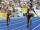Two runners ran simultaneously towards each other from locations distant 34.6 km. The average speed of the first runner was 1/5 higher than the average speed of the second runner. How long should each ran a 34.6 km, if you know that they meet after 67 min
• Against each other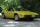From two points A, B distant 23 km at the same time started two cars against each other at speeds 41 km/h and 65 km/h. How long does cars meet and what distance passes each of them?
• Double-track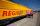On double-track express met with a freight train. Express travel at speed 72 km/h, a freight train at speed 36 km/h. After what time period will be from 9 km apart?